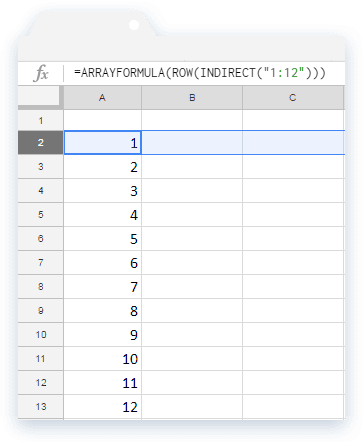# How to use the ROW formula in Google SheetsThe ROW formula is one of the lookup functions available within Google Sheets. It gives us the row number where the specified cell or a range of cells are located.

### Syntax

ROW([cell_reference])

cell_reference – is the address reference to the cell whose row number we need. This is an optional parameter. So, if not specified, the ROW formula considers the address of the cell where we keyed in the formula.

Please note that if the cell_reference parameter is not a single cell, but is a range of multiple cells, the formula returns only the row number of the first row within the cell_reference.

### Usage: ROW Formula

Let us get our understanding of the formula more concrete by means of a few examples. Please see the snapshot below.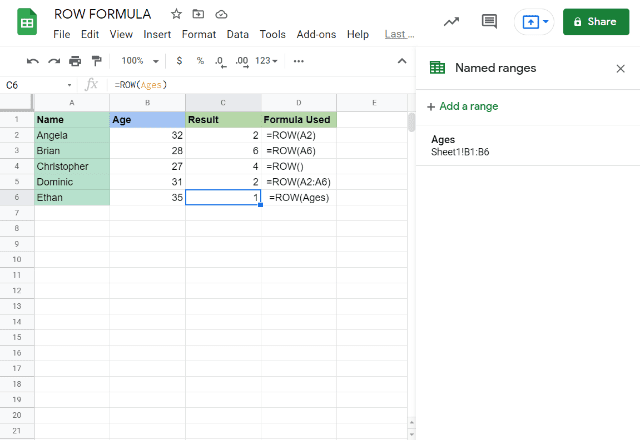The first example took A2 for cell_reference and accordingly returned 2 as output. Similarly, for A6, it returned 6 as output for the second example. The third case doesn’t have any input for the cell_reference, hence it returned the row number of the cell in which we keyed in the formula.

In the fifth example, we provided an address to a range of cells as opposed to a single cell. And, it returned the number of the first row within the range A2:A6, which is 2.

It is interesting to note that we provided a named range reference as input for the cell_reference parameter in the last example case. As shown on the extreme right of the above image, the “Ages” range spans from B1 to B6. Therefore, the result is the first row number of range B1:B6.

##### Use case: Auto-filling numbers

One of the useful ways we can use the ROW formula is when we use it in conjunction with the ARRAYFORMULA. We use the following method to have Google Sheets fill up series of numbers for us without having to enter them ourselves.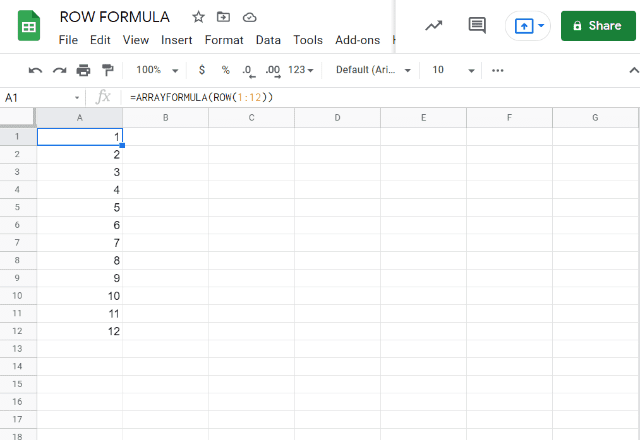This utility of the formula is very handy indeed. Even if we try and insert a row anywhere between the existing rows, the numbering automatically is taken care of. We will insert a row between rows 6 and 7 as shown below. And we will notice we don’t have to do anything to adjust the numbering – Google Sheets does that for us automatically.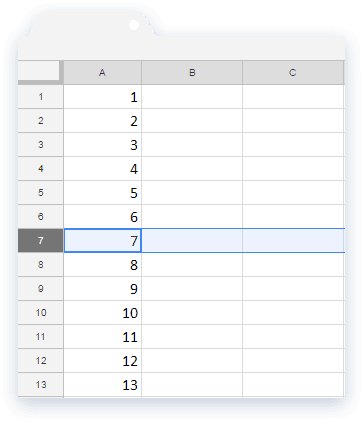But then, there is a small problem. What if we try inserting a row above the cell A1? Doing so would shift the cell down and accordingly adjusts the formula as shown below, which is probably undesirable.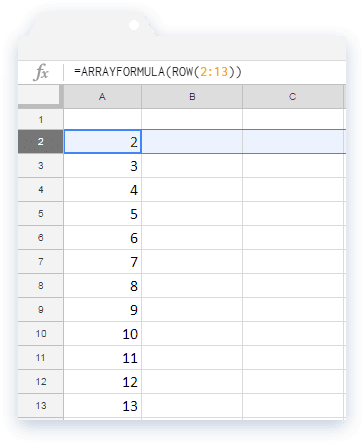We can fix this issue using the INDIRECT formula as shown below. Even if we insert a row above, it doesn’t affect the numbering. However, the address reference 1:12 has now become static. So, inserting rows between 1 through 12 will not adjust the reference to 1:13 anymore (like it did in the third snapshot).